Lecture 14
Correlation

Association is one of the fundamental tools of scientists. Francis Bacon, for instance, discovered that heat is a form of motion by compiling lists of items that were hot and cold. Ivan Pavlov, who was originally studying the digestive system, discovered an important rule of learning, classical conditioning, by observing that dogs salivated when he rang their dinner bell. In both instances, an association was noted between two variables. As one variable increases, so does the other.

The statistical index of the degree to which two variables are associated is the correlation coefficient. Developed by Karl Pearson, it is sometimes called the "Pearson correlation coefficient". The correlation coefficient summarizes the relationship between two variables.

Let's take an example. Did you ever wonder whether the person that took the longest on the test did very well or very poorly? It might be that the students who take the longest on the exam are the most careful, and they score the highest. This would be an example of a positive correlation, because high values of one variable (e.g., time spent on the test) are associated with high values on the other variable (e.g., better performance on the test). Or it might be the other way around: longer time on the test is associated with poorer scores. The latter is an example of a negative correlation, because high values on one variable are associated with low values on another variable. A person who scores highly usually finishes quickly.

To examine whether there is a positive or negative association between grades on an exam and time spent on an exam, one has to look to see if individuals who did well on the exam also spent longer on it. Here are some hypothetical grades on an exam and the amount of time each student spent on the exam.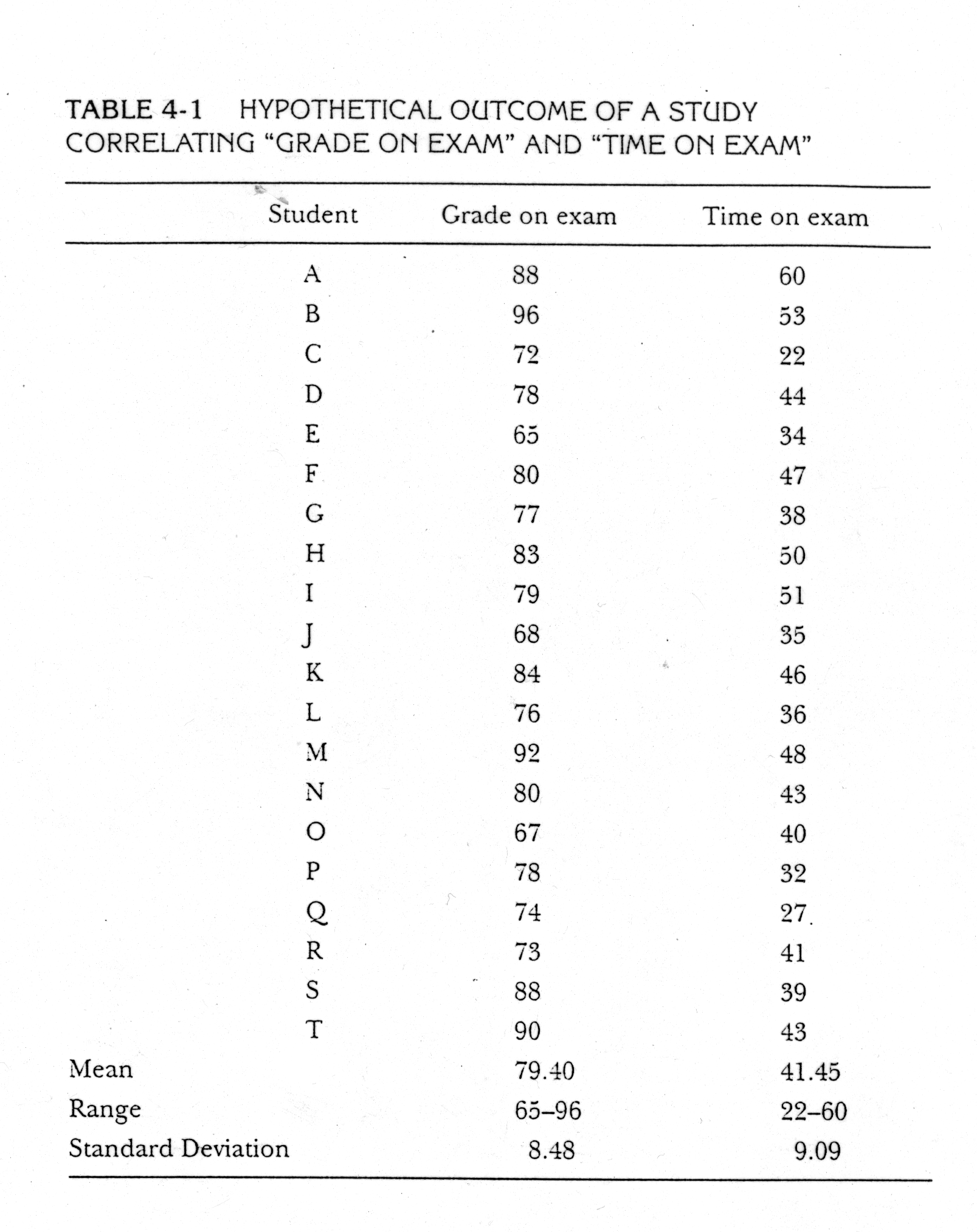One way to find out if there is a positive or negative relationship is to examine the list and see if the highest grades are associated with the shortest or longest time spent on the exam. But it is difficult to easily see if there is a relationship between the variables this way. A better method is to create a bivariate scatter plot (bivariate meaning two variables). If we plot the scores from the table above with the time on the x-axis and the grades on the y-axis, we would get something that looks like this: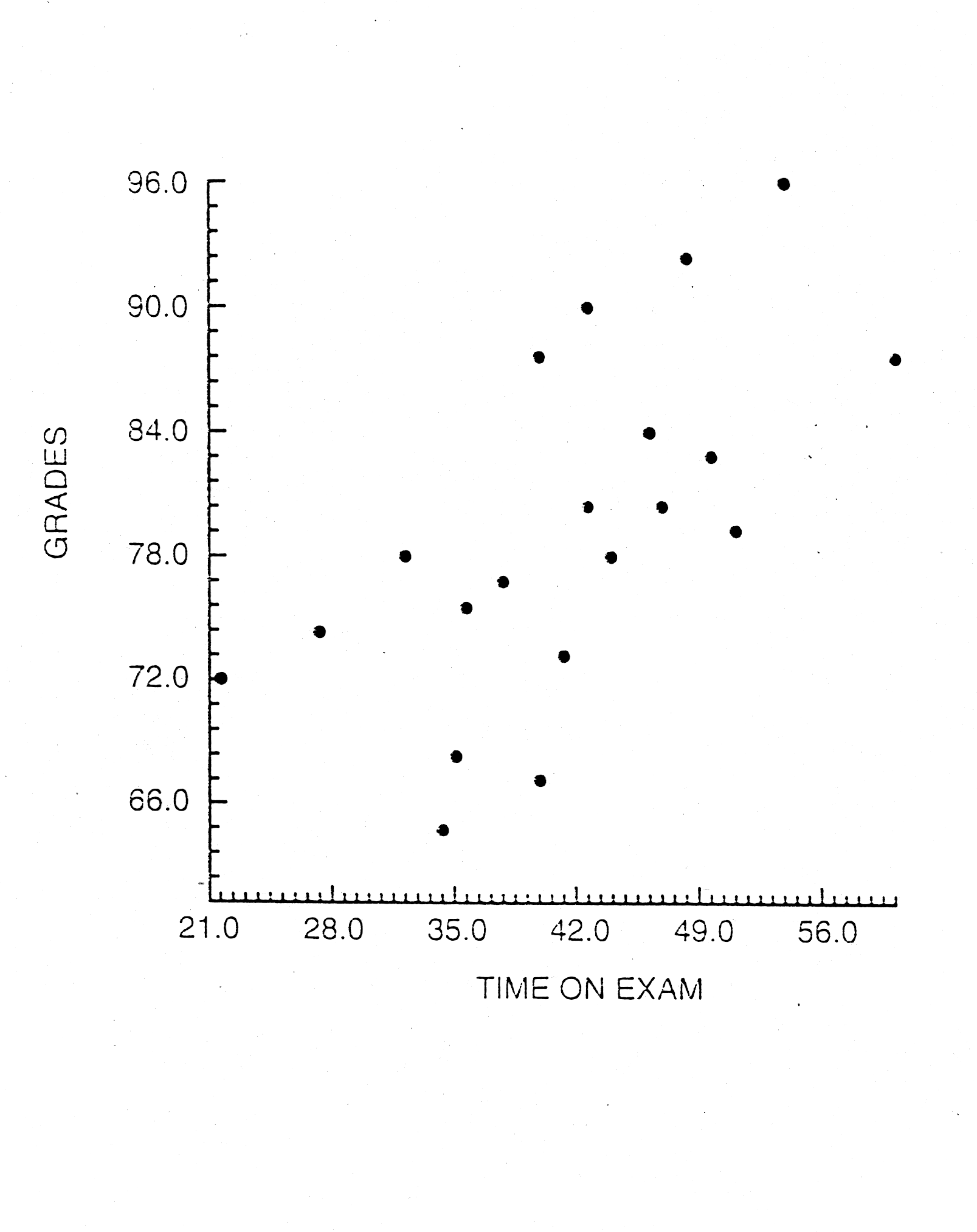Each point represents one student with a certain score for time on the exam, x, and grade, y. The scatter plot reveals that, in general, longer times on the exam tend to be associated with higher grades. Notice that there is a kind of stream of points moving from the bottom left hand corner of the graph to the upper right hand corner. That indicates a positive association or correlation between the two variables.

As always, we have a letter that stands for out statistic. In the case of correlation, it is r. The Pearson r can be positive or negative, ranging from -1.0 to 1.0. A correlation of 1.0 indicates a perfect positive association between the two variables. If the correlation is 1.0, the longer the amount of time spent on the exam, the higher the grade will be--without any exceptions. An r value of -1.0 indicates a perfect negative correlation--without an exception, the longer one spends on the exam, the poorer the grade. If r=0, there is absolutely no relationship between the two variables. When r=0, on average, longer time spent on the exam does not result in any higher or lower grade. Most often r is somewhere in between -1.0 and +1.0.

Take a minute to look at some examples of scatter plots with different correlations, by clicking here.

In these graphs, the r values are in parentheses. Notice that for the perfect correlation, there is a perfect line of points. They do not deviate from that line. For moderate values of r, the points have some scatter, but there still tends to be an association between the x and the y variables. When there is no association between the variables, the scattering is so great that there is no discernable pattern.

Correlations can be said to vary in magnitude and direction. Magnitude refers to the strength of association--higher r values represent stronger relationship between the two variables. Direction refers to whether the relationship is positive or negative, and hence the value of r is positive or negative.

One can think of a correlation as measure the degree of overlap, or how much two variables tend to vary together. Go back to the scatter plot printed above, and put your hand over the y-axis (vertical one!!). How much the points vary from left to right is how much variation there is in the time variable. Now, put your hand over the x-axis. Look at how much the points vary from top to bottom. That amount of scatter represents the variation in grades. Now, looking at the bivariate plot as whole, you can see how the points tend to scatter or vary together. Their "shared variance" is the amount that the variations of the two variables tend to overlap.

The percentage of shared variance is represented by the square of the correlation coefficient, r2. Another way to visualize this is with a Venn diagram that represents the amount of shared variance, or overlap of variation, of two variables. Click here to see some examples. Because r-square is interpreted as the percentage of shared variance, it is best to compare two r2s rather than two rs. For instance, a correlation of .8 seems to be twice as large as a correlation of .4. But the larger coefficient actually indicates there is 4 times as much shared variance. .64 vs. .16. Occasionally, shared variance is called the variance accounted for in one variable by another variable. An r-square of .64 suggests that x accounts for 64% of the variance in y.

Example
Let's look quickly at an example using the grade and time study above. (My apologies for switching the x and y in this example).

 ID Grade on Exam (x) x2 Time on Exam (y) y2 xy 1 88 7744 60 3600 5280 2 96 9216 53 2809 5088 3 72 5184 22 484 1584 4 78 6084 44 1936 3432 5 65 4225 34 1156 2210 6 80 6400 47 2209 3760 7 77 5929 38 1444 2926 8 83 6889 50 2500 4150 9 79 6241 51 2601 4029 10 68 4624 35 1225 2380 11 84 7056 46 2116 3864 12 76 5776 36 1296 2736 13 92 8464 48 2304 4416 14 80 6400 43 1849 3440 15 67 4489 40 1600 2680 16 78 6084 32 1024 2496 17 74 5476 27 729 1998 18 73 5329 41 1681 2993 19 88 7744 39 1521 3432 20 90 8100 43 1849 3870 S 1588 127454 829 35933 66764

The formula for correlation is really just a computational one. It does not make much sense as is, but will give us a correlation coefficient more quickly.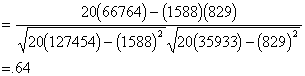To test r for significance, we test the null hypothesis that, in the population, the correlation is zero. To do that we compute a t statistic.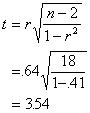The d.f. for the test is n - 2 =18, and we use the usual t table. The critical value is 2.101 at alpha=.05, so the correlation is significantly greater than zero. In other words, there is a statistically significant linear relationship between the grades and time spent on the exam. If we were to measure exams grades and time spent on test in the population, we expect that the correlation between the two would be greater than 0.

Standardized Relationship
The Pearson r can be thought of as a standardized measure of the association between two variables. That is, a correlation between two variables equal to .64 is the same strength of relationship as the correlation of .64 for two entirely different variables. The metric by which we gauge associations is a standard metric.

Also, it turns out that correlation can be thought of as a relationship between two variables that have first been standardized or converted to z scores.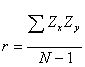Correlation Represents a Linear Relationship
Correlation involves a linear relationship. "Linear" refers to the fact that, when we graph our two variables, and there is a correlation, we get a line of points. In an algebraic sense, linear refers to the fact that we can add, subtract, multiply, or divide one of the variables by a number to get an approximation of the other variable. (If you don't get this last explanation, don' t worry, we'll revisit it later).

Correlation tells you how much two variables are linearly related, not necessarily how much they are related in general. It is true that the most common measure of association is correlation, and, hence, whether or not there is a relationship is usually determined by whether or not there is a correlation. However, there are exceptions. A curvilinear relationship is one example. In some cases, two variables may have a strong, or even perfect, relationship, yet the relationship is not at all linear. In these cases, the correlation coefficient might be zero. Take for example, a well know psychological relationship between arousal and performance. This is referred to as the Yerkes-Dobson law. If someone has very low arousal (e.g. half-asleep), performance on a test will be very poor. If one is moderately aroused, the performance on the test will be high because of stronger motivation. If that arousal becomes too high, as it is with extreme test anxiety, performance on an exam will be very poor. So, overall, there is not a linear relationship between arousal and performance, because there is no general tendency to do better as arousal increases.

Here is a graph of the Yerkes-Dobson curve, in which the correlation between arousal and performance is zero, but there is a strong curvilinear relationship.

The best way to make sure that your correlation coefficient is not misleading about the relationship between the two variables is to look at a bivariate plot.

Restricted range
Correlations can be deceiving if the full information about each of the variables is not available. A correlation between two variables is smaller if the range of one or both variables is truncated. This is called the restricted range phenomenon. The range of one or both of the variables is restricted or truncated. Because the full variation of one of the variables is not available, there is not enough information to see the two variables covary together.

These graphs illustrate restricted range.

So, sometimes a small or zero correlation may obtained, because of restricted range rather than because there is not really a true relationship between the two variables.